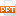# Bài giảng An Introduction to Computer Science Using Java - Chapter 6 Iteration

Tài liệu Bài giảng An Introduction to Computer Science Using Java - Chapter 6 Iteration: Chapter 6 IterationLecture Slides to AccompanyAn Introduction to Computer Science Using Java (2nd Edition)byS.N. Kamin, D. Mickunas, E. ReingoldChapter PreviewIn this chapter we will:discuss the use of repetition (iteration) in programming algorithmsdescribe the Java while, for, and do-while statementsdemonstrate the use of loop invariants to verify the correctness of loopsshow the use of loops in data entry and computer animation while LoopRepeated executes body of the loop which can be a single or compound statementA while loop will execute as long as its condition is trueAn infinite loop will occur if the condition never becomes falseExample: count = 1; while (count 0) { rest = rest / 10; numberOfDigits++; };Reading Input Using a LoopDone using do-whileread scoreif ( not end of input)do { process score read score } while ( not end of input ); Done using whileread scorewhile ( not end of input ) { process score read score};Sentinel Controlled Loopint i = 0;InputBox in = new InputBox(...20 trang | Chia sẻ: honghanh66 | Ngày: 19/03/2018 | Lượt xem: 175 | Lượt tải: 0
Bạn đang xem nội dung tài liệu Bài giảng An Introduction to Computer Science Using Java - Chapter 6 Iteration, để tải tài liệu về máy bạn click vào nút DOWNLOAD ở trên
Chapter 6 IterationLecture Slides to AccompanyAn Introduction to Computer Science Using Java (2nd Edition)byS.N. Kamin, D. Mickunas, E. ReingoldChapter PreviewIn this chapter we will:discuss the use of repetition (iteration) in programming algorithmsdescribe the Java while, for, and do-while statementsdemonstrate the use of loop invariants to verify the correctness of loopsshow the use of loops in data entry and computer animation while LoopRepeated executes body of the loop which can be a single or compound statementA while loop will execute as long as its condition is trueAn infinite loop will occur if the condition never becomes falseExample: count = 1; while (count 0) { rest = rest / 10; numberOfDigits++; };Reading Input Using a LoopDone using do-whileread scoreif ( not end of input)do { process score read score } while ( not end of input ); Done using whileread scorewhile ( not end of input ) { process score read score};Sentinel Controlled Loopint i = 0;InputBox in = new InputBox();In.setPrompt(“Enter data or –1 to terminate”);// read first scorescore = in.readInt();while ( score != -1 ) { i++; // insert code to process score // read next score score = in.readInt();}Reading to End of Inputint i = 0;InputBox in = new InputBox();In.setPrompt(“Enter score (empty input ends data”);// read first scorescore = in.readInt();while ( !in.eoi() ) { i++; // insert code to process score // read next score score = in.readInt();}Loop-and-a-HalfDone using whileread scorewhile ( !in.eoi( ) ) { process score read score}; Done using do-whiledo { read score if ( !in.eoi( ) ) process score} while ( !in.eoi( ) );Infinite Loop with EscapeDone using do-whiledo { read if ( in.eoi( ) ) return process} while ( true ); Done using whilewhile (true) { read if ( in.eoi( ) ) return process score};Drawing in Java// code to read and display GIF image from fileimport java.awt.*;Import CSLib.*;DrawingBox g = new DrawingBox();Toolkit tools = Toolkit.getDefaultToolkit():Image mouse = tools.getImage(“C:/KMR/images/mouse.gif”);g.drawImage(mouse, x, y);Nested Loopsint advance = 0;// Draw clock and read input repeatedlydo { for (int i = 1; i <= advance; i++) { // Advance time c.setMinute(c.getMinute()+1); // Update clock display d.clear(); c.display(d, SIZE/2, SIZE/2, SIZE/2); } in.setPrompt(“Advance how many minutes?”); advance = in.readInt();} while (!in.eoi());

Các file đính kèm theo tài liệu này:

•chapter6_3674.ppt
Tài liệu liên quan